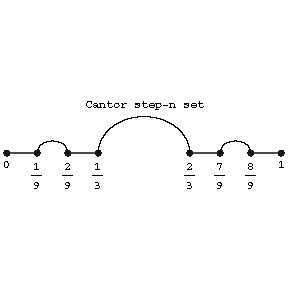# Cantor set

The subset of the real interval $[0,1]$ consisting of all numbers of the form $\sum_{i=1}^\infty\epsilon_i/3^i$, where $\epsilon_i$ is 0 or 2. It is geometrically described as follows (see Fig.): One removes from $[0,1]$ its middle third $(1/3,2/3)$; one then removes from the remaining intervals $[0,1/3]$, $[2/3,1]$ their middle thirds $(1/9,2/9)$ and $(7/9,8/9)$; then the middle thirds of the four remaining intervals, etc. Then what remains after removal of all these intervals (adjacent intervals), the total of whose length is 1, is the Cantor perfect set (Cantor set; Cantor ternary set; Cantor discontinuum).

It is nowhere dense in the real line but has the cardinality of the continuum.Figure: c020250a

From a topological point of view, the Cantor set is a zero-dimensional, perfect, metrizable compactum (that is, without isolated points); such a compactum is unique up to a homeomorphism. All bounded, perfect, nowhere-dense subsets of the real line are similar sets. The Cantor set is homeomorphic to a countable product $D^{\aleph_0}$ of copies of a two-point discrete space $D$, and is the space of the topological group $\mathbf Z_2^{\aleph_0}$. The Cantor set is universal in two senses: 1) first of all, every zero-dimensional regular Hausdorff space with a countable base is homeomorphic to a subset of the Cantor set; 2) secondly, every metrizable compactum is a continuous image of the Cantor set (Aleksandrov's theorem). This theorem marks the beginning of the theory of dyadic compacta and shows that many compacta are similar to one another from the functional point of view. In particular, all perfect compacta have the same Boolean algebra of canonical open sets. The existence of special mappings from the Cantor set onto compacta allows one to prove that the Banach algebras of all continuous functions on two arbitrary perfect metrizable compacta (for example, on an interval and on a square) are linearly homeomorphic. Furthermore, the Cantor set and the possibility of mapping it onto an arbitrary metrizable compactum lies at the basis of the construction of many interesting examples in topology and function theory. One of them is the so-called Cantor staircase, which is the graph of a continuous monotone mapping of $[0,1]$ onto itself, the derivative of which is defined and equal to zero on an open set of measure 1. Although the standard Cantor set has measure zero, there exists nowhere-dense perfect compacta on the unit interval with measure arbitrarily close to 1.

How to Cite This Entry:
Cantor set. Encyclopedia of Mathematics. URL: http://encyclopediaofmath.org/index.php?title=Cantor_set&oldid=38566
This article was adapted from an original article by V.V. Fedorchuk (originator), which appeared in Encyclopedia of Mathematics - ISBN 1402006098. See original article